# Analytical reasoning test-2

21)

In each of the the following question, count the number of triangles and squares in the given figure.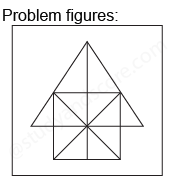22)

How many triangles and parallelograms are there in the following figure?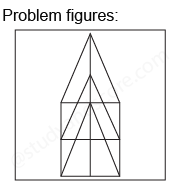23)

How many rectangles are there in the following figure.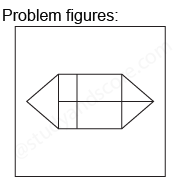24)

In each of the following question, count the number of parallelogram in the given figure.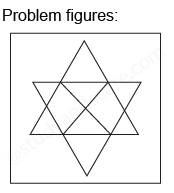25)

What is the minimum number of straight lines that is needed to construct the figure?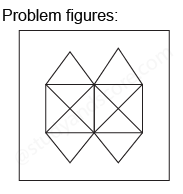--Share with your friends --